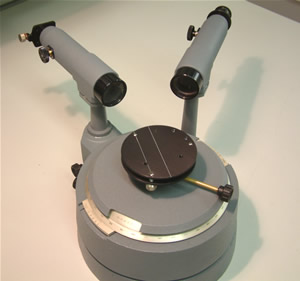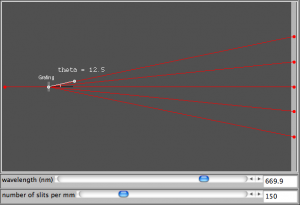# diffraction gratings

We have used the grating equation$n \lambda = d \sin \theta$

to measure the wavelength of light produced by a laser.  This equation is also useful to predict the location of bright fringes produced by a diffraction grating.  Remember that d in this equation is the distance between adjacent lines in the grating and not the number of lines per metre/millimetre. More about d on this A-level revision page.

We’ll look at applications of this equation a little more this week, e.g. using a spectrometer to measure the angle so we can calculate the wavelength of the light used.###### spectrometer [photo by mrmackenzie]

In the meantime, get some practice at using the grating equation with the simulation site shown below.  You can select how many lines you would like per millimeter of grating and alter the wavelength.  Try calculating the angle for the first or second order spots and then use the simulated protractor to see if you are correct.

Click on the image below to get started.You need to have Java installed to run the simulation.

I have attached a pdf with some notes on diffraction gratings.  Read through them and make sure you are happy with the theory so you are able to complete the experiments in class.

This site uses Akismet to reduce spam. Learn how your comment data is processed.